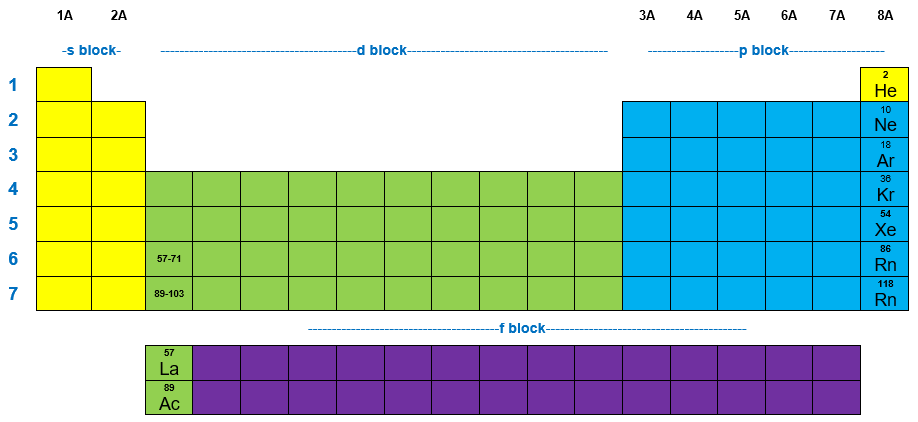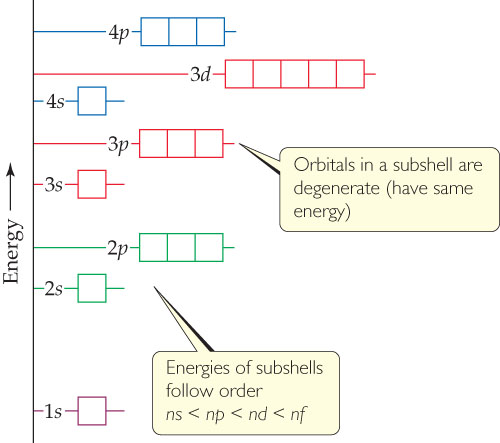# Problem: General energy ordering of orbitals for a many-electron atom.Not all of the orbitals in the n exttip{n}{n}= 4 shell are shown in this figure. Which subshells are missing?

###### FREE Expert Solution

We’re being asked to determine which subshells from n = 4 are missing from the given diagram.

Let’s refer to the illustration of the periodic table below:95% (282 ratings)###### Problem DetailsGeneral energy ordering of orbitals for a many-electron atom.

Not all of the orbitals in the n = 4 shell are shown in this figure. Which subshells are missing?